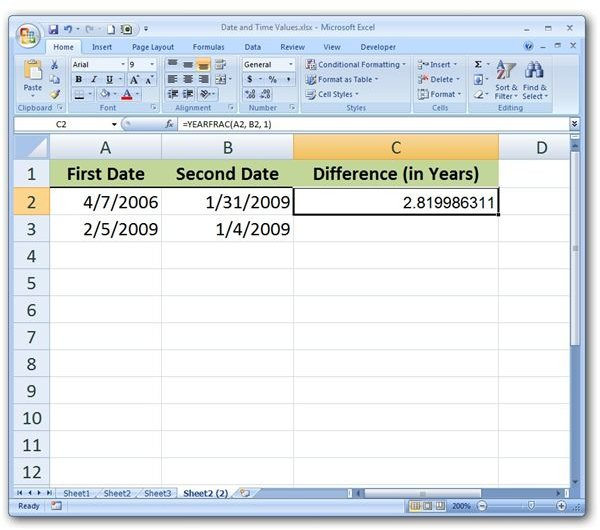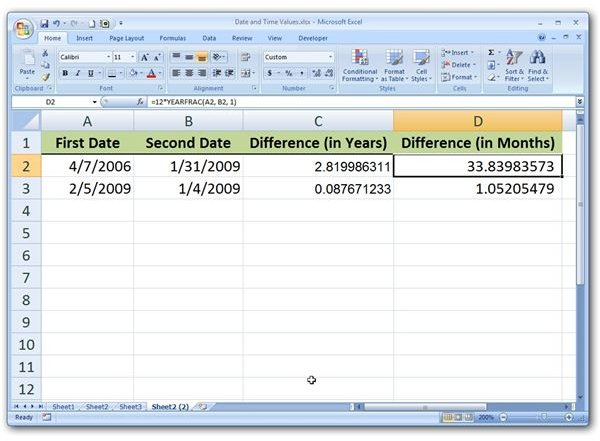# Calculate the Partial Year Difference between Two Dates with the YEARFRAC Function in Microsoft Excel

Page content

## Calculating Precise Differences in Dates

In Subtracting Dates in Microsoft Excel, we looked at a basic way to find the difference between two dates in Microsoft Excel. That method works well for the times when you only want to know the number of days between two dates, but it has some limitations if you want to calculate the difference in terms of years or months. For cases when we want to find more precise information, including those times we want the result to reflect partial years and months, it’s better to use the YEARFRAC function.

## Syntax of YEARFRAC Function

The syntax for the YEARFRAC function is

YEARFRAC(start_date, end_date, basis)

where start_date and end_date represent the two dates you want to find the difference between and basis refers to the method you want to use when determining how many days are in a month or year. The following values can be used for the basis argument.

• 0 – This value is used for US (NASD) date systems that assume 30 days are in a month and 360 days are in one year. If no basis value is specified, a value of 0 is used.

• 1 – A value of 1 should be used for the basis if you want your calculations based on the actual number of days in any given month or year.

• 2 – This value uses the actual number of days in any given month but assumes there are only 360 days in a year.

• 3 – This value is similar to the previous option as it uses the actual number of days in any given month, but it assumes there are 365 days in each year. That is, it disregards leap years.

• 4 – This value is similar to 0 (30 days in each month and 360 days in a year), but it assumes a European date system.

## YEARFRAC Function in Use

The nice thing about the YEARFRAC function is that it takes partial years into account. The best way to explain this further is to look at an example. Suppose we want to calculate the number of years between the dates shown in Column A and Column B in the spreadsheet below. (Click any image for a larger view.)

In this example, our start_date is 4/7/2006 (cell A2) and our end_date is 1/31/2009 (cell B2). We’ll use a basis value of 1 in order to calculate the true difference between the two dates. Our function can be stated as:

=YEARFRAC(A2, B2, 1)Note that the order of the dates doesn’t matter. Even if the so-called start_date is more recent than the end_date, the YEARFRAC function will still return a positive value.

What if you want the difference to be stated in terms of months instead of years? In this case, all you have to do is multiply the result by 12 (the number of months in a year).

=12 * YEARFRAC(A2, B2, 1)If you want the final answer to be represented in terms of days, it’s still better to use the basic subtraction method described in Subtracting Dates in Microsoft Excel (see the link to this article in the first section).

For more tips and tricks, browse through Bright Hub’s library of Microsoft Excel tutorials and user guides. Learn about lookup and reference functions, how to create charts and graphs, and more. Additional articles are being added on a regular basis, so check back often.